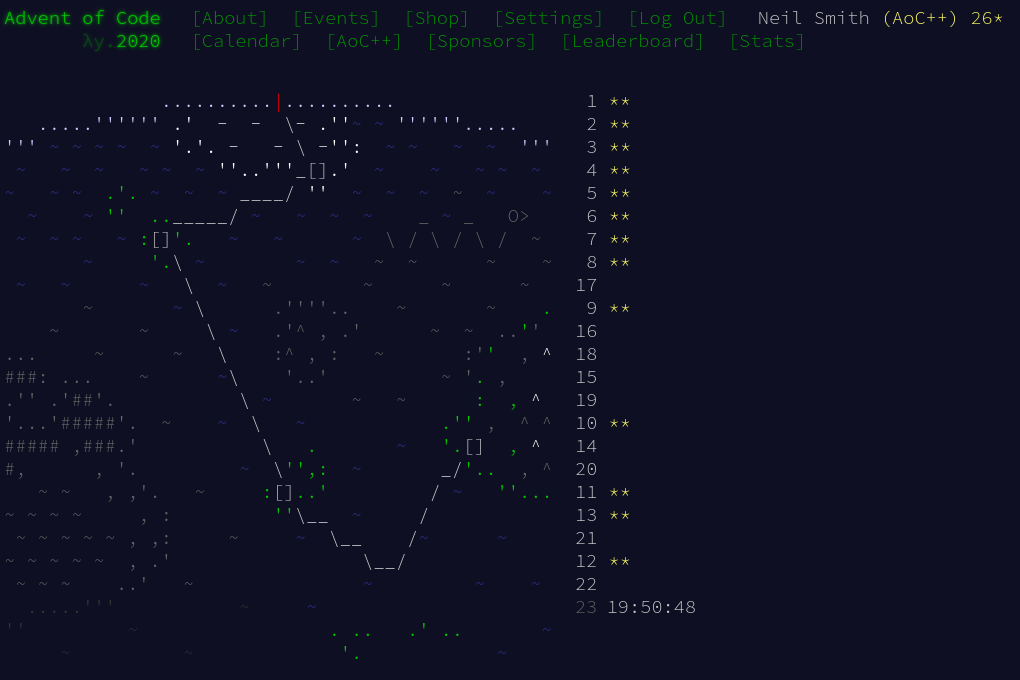December 22, 2020

# Advent of Code 2020 day 12Day 12 was fairly straighforward: apply a bunch of rules to update a state. The two parts are so similar, I'll treat them together here.

I started with some data definitions, for Directions, the Actions and the Ship.

data Direction = North | East | South | West
deriving (Show, Eq, Ord, Enum, Bounded)

type Position = (Int, Int) -- (x, y)

data Action a = N a | S a | E a | W a | L a | R a | F a
deriving (Show, Eq, Ord)

data Ship = Ship { position :: Position
, direction :: Direction
, waypoint :: Position
}
deriving (Show, Eq, Ord)


I thought about using complex numbers for the position, but decided that the benefit wasn't worth it: I'd be spending most of my time looking at the components of the position, so there was no real benefit in bundling them together.

Direction is a Bounded Enum so that I can increase and decrease it. It's time to reuse my "wrapping enum" definitions, so "increasing" the direction turns clockwise, and "increasing" from West correctly turns North.

-- | a succ that wraps
succW :: (Bounded a, Enum a, Eq a) => a -> a
succW dir | dir == maxBound = minBound
| otherwise = succ dir

-- | a pred that wraps
predW :: (Bounded a, Enum a, Eq a) => a -> a
predW dir | dir == minBound = maxBound
| otherwise = pred dir


Parsing the inputs is explicit: one rule for each action, a general action is a choice of the specific rules.

actionsP = actionP sepBy endOfLine

actionP = choice [nP, sP, eP, wP, lP, rP, fP]

nP = N <$> ("N" *> decimal) sP = S <$> ("S" *> decimal)
eP = E <$> ("E" *> decimal) wP = W <$> ("W" *> decimal)
lP = L <$> ("L" *> decimal) rP = R <$> ("R" *> decimal)
fP = F <\$> ("F" *> decimal)


Applying the actions is a fold, where each step in the fold has type act :: Ship -> Action Int -> Ship.

part1 actions = manhattan (position ship1) start
where start = (0, 0)
ship0 = Ship {position = start, direction = East, waypoint = (10, 1)}
ship1 = foldl act ship0 actions

part2 actions = manhattan (position ship1) start
where start = (0, 0)
ship0 = Ship {position = start, direction = East, waypoint = (10, 1)}
ship1 = foldl actW ship0 actions


All that's needed is to apply each action to a ship. Note the use of record wildcards to help packing and unpacking the record. For part 1, it looks like this:

act :: Ship -> Action Int -> Ship
act Ship{..} (N d) = Ship { position = dDelta d North position, ..}
act Ship{..} (S d) = Ship { position = dDelta d South position, ..}
act Ship{..} (W d) = Ship { position = dDelta d West  position, ..}
act Ship{..} (E d) = Ship { position = dDelta d East  position, ..}
act Ship{..} (L a) = Ship { direction = d, ..} where d = (iterate predW direction) !! (a div 90)
act Ship{..} (R a) = Ship { direction = d, ..} where d = (iterate succW direction) !! (a div 90)
act Ship{..} (F d) = Ship { position = dDelta d direction position, ..}


For part 2, the actW (for "waypoint") looks like this:

actW :: Ship -> Action Int -> Ship
actW Ship{..} (N d) = Ship { waypoint = dDelta d North waypoint, ..}
actW Ship{..} (S d) = Ship { waypoint = dDelta d South waypoint, ..}
actW Ship{..} (W d) = Ship { waypoint = dDelta d West  waypoint, ..}
actW Ship{..} (E d) = Ship { waypoint = dDelta d East  waypoint, ..}
actW Ship{..} (L a) = Ship { waypoint = d, ..} where d = (iterate rotL waypoint) !! (a div 90)
actW Ship{..} (R a) = Ship { waypoint = d, ..} where d = (iterate rotR waypoint) !! (a div 90)
actW Ship{..} (F d) = Ship { position = p', ..}
where (x, y) = position
(dx, dy) = waypoint
p' = (x + (d * dx), y + (d * dy))


Most of the actions involve moving a position by a particular distance in a particular direction, so I wrote a utility function to do that.

delta North = ( 0,  1)
delta South = ( 0, -1)
delta East  = ( 1,  0)
delta West  = (-1,  0)

dDelta dist dir (x, y) = (x + (dist * dx), y + (dist * dy))
where (dx, dy) = delta dir


## Code

You can find the code here or on Gitlab.